# Modular lattice

(diff) ← Older revision | Latest revision (diff) | Newer revision → (diff)

Dedekind lattice

A lattice in which the modular law is valid, i.e. if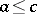, thenfor any. This requirement amounts to saying that the identityis valid. Examples of modular lattices include the lattices of subspaces of a linear space, of normal subgroups (but not all subgroups) of a group, of ideals in a ring, etc. A lattice with a composition sequence is a modular lattice if and only if there exists on it a dimension function, i.e. an integer-valued function such that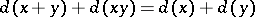and such that if the intervalis prime, it follows that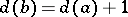. If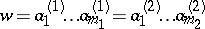, if none of the elementscan be represented as a product of elements other than itself and if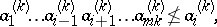then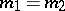and for any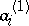it is possible to find an element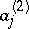such that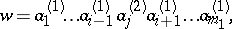, . Non-zero elementsof a modular lattice with a zero 0 are said to be independent if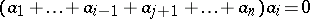for all. This definition makes it possible to generalize many properties of systems of linearly independent vectors , , . Ifare independent, their sum is denoted by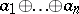. Ore's theorem: If a modular lattice has a composition sequence and if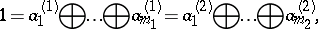none of the elementsbeing representable as a sum of two independent elements, thenand for eachit is possible to find an elementsuch that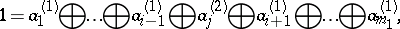, . In the case of completely modular lattices (cf. also Complete Dedekind lattice), which must satisfy certain additional requirements, the theorems on independent elements and direct decompositions may be applied to infinite sets as well , . Complemented modular lattices have been studied; these are modular lattices with a 0 and a 1 in which for each elementthere exists at least one element(said to be a complement of the element) such that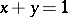,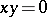. A complemented modular lattice which has a composition sequence, is isomorphic to the modular lattice of all subspaces of a finite-dimensional linear space over some skew-field. A complemented completely modular latticeis isomorphic to the modular lattice of all subspaces of a linear (not necessarily finite-dimensional) space over some skew-field if and only if the following conditions are met: a) if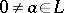, it is possible to find an atom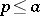; b) ifis an atom and, where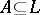, then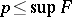for some finite set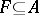; c) if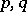are distinct atoms, it is possible to find a third atom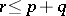; and d) there exist at least three independent atoms. The last condition d) may be replaced by the requirement that the Desargues assumption be valid . A further generalization of this result, which leads to regular rings , , is connected with the theory of von Neumann algebras. For a modular lattice with a composition sequence the presence of complements is equivalent to the representability of the unit as a sum of atoms.

Modular lattices are (in the Soviet Union) also called Dedekind lattices, in honour of R. Dedekind, who was the first to formulate the modular law and established a number of its consequences .

How to Cite This Entry:
Modular lattice. Encyclopedia of Mathematics. URL: http://encyclopediaofmath.org/index.php?title=Modular_lattice&oldid=11893
This article was adapted from an original article by L.A. Skornyakov (originator), which appeared in Encyclopedia of Mathematics - ISBN 1402006098. See original article